# Iterating over a Dictionary in Swift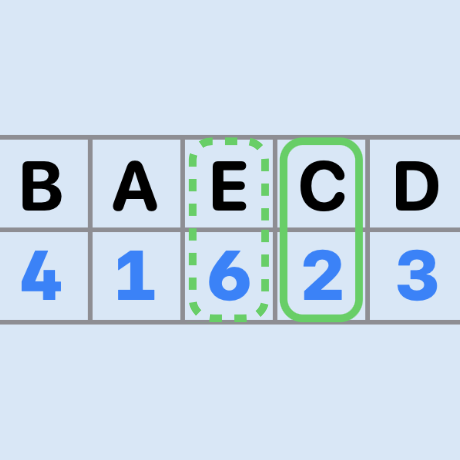A dictionary stores a list of key/value pairs in an unordered structure that is fast and efficient at adding elements and finding elements by a key. Each element in the dictionary is identified using its key, which is a hashable type such as a string or number.

## Looping over Dictionary elements

Start with the default for looping through a dictionary. Create a dictionary with keys of type String and values of integers. Note that printing the dictionary does not print the elements in the order they were added to the dictionary. The dictionary is an unordered collection of key/value pairs that allows fast access to elements by key. A for loop can be used to iterate over the elements and perform functions with the elements, this code just prints out the elements.

 1let dict = ["red": 23,
2           "orange": 10,
3           "yellow": 87,
4           "green": 39,
5           "blue": 21,
6           "indigo": 92,
7           "violet": 56]
8
9print(dict)
10print()
11
12for item in dict {
13   print(item)
14}
15
16"""
17["indigo": 92, "red": 23, "green": 39, "blue": 21, "violet": 56, "orange": 10, "yellow": 87]
18
19(key: "indigo", value: 92)
20(key: "red", value: 23)
21(key: "green", value: 39)
22(key: "blue", value: 21)
23(key: "violet", value: 56)
24(key: "orange", value: 10)
25(key: "yellow", value: 87)
26"""


## Loop with forEach

A ForEach loop can be used directly on the Dictionary to yield the same results as the For loop. The Map method can also be used with the print function to iterate over the elements and print them out.

 1dict.forEach { print($0) } 2print() 3 4dict.map { print($0) }
5print()
6
7"""
8(key: "indigo", value: 92)
9(key: "red", value: 23)
10(key: "green", value: 39)
11(key: "blue", value: 21)
12(key: "violet", value: 56)
13(key: "orange", value: 10)
14(key: "yellow", value: 87)
15
16(key: "indigo", value: 92)
17(key: "red", value: 23)
18(key: "green", value: 39)
19(key: "blue", value: 21)
20(key: "violet", value: 56)
21(key: "orange", value: 10)
22(key: "yellow", value: 87)
23"""


Each element in the for loop is a Tuple of key and value that can be named explicitly and referenced in the loop. In the ForEach or Map syntax the elements of the Tuple can be accessed by index using dot notation.

 1for (color,num) in dict {
2   print("The value for color \(color) is \(num)")
3}
4print()
5
6dict.forEach {
7   print("The value for color \($0.0) is \($0.1)")
8}
9print()
10
11dict.map {
12   print("The value for color \($0.0) is \($0.1)")
13}
14print()
15
16"""
17The value for color red is 23
18The value for color orange is 10
19The value for color green is 39
20The value for color yellow is 87
21The value for color violet is 56
22The value for color indigo is 92
23The value for color blue is 21
24
25The value for color red is 23
26The value for color orange is 10
27The value for color green is 39
28The value for color yellow is 87
29The value for color violet is 56
30The value for color indigo is 92
31The value for color blue is 21
32
33The value for color red is 23
34The value for color orange is 10
35The value for color green is 39
36The value for color yellow is 87
37The value for color violet is 56
38The value for color indigo is 92
39The value for color blue is 21
40"""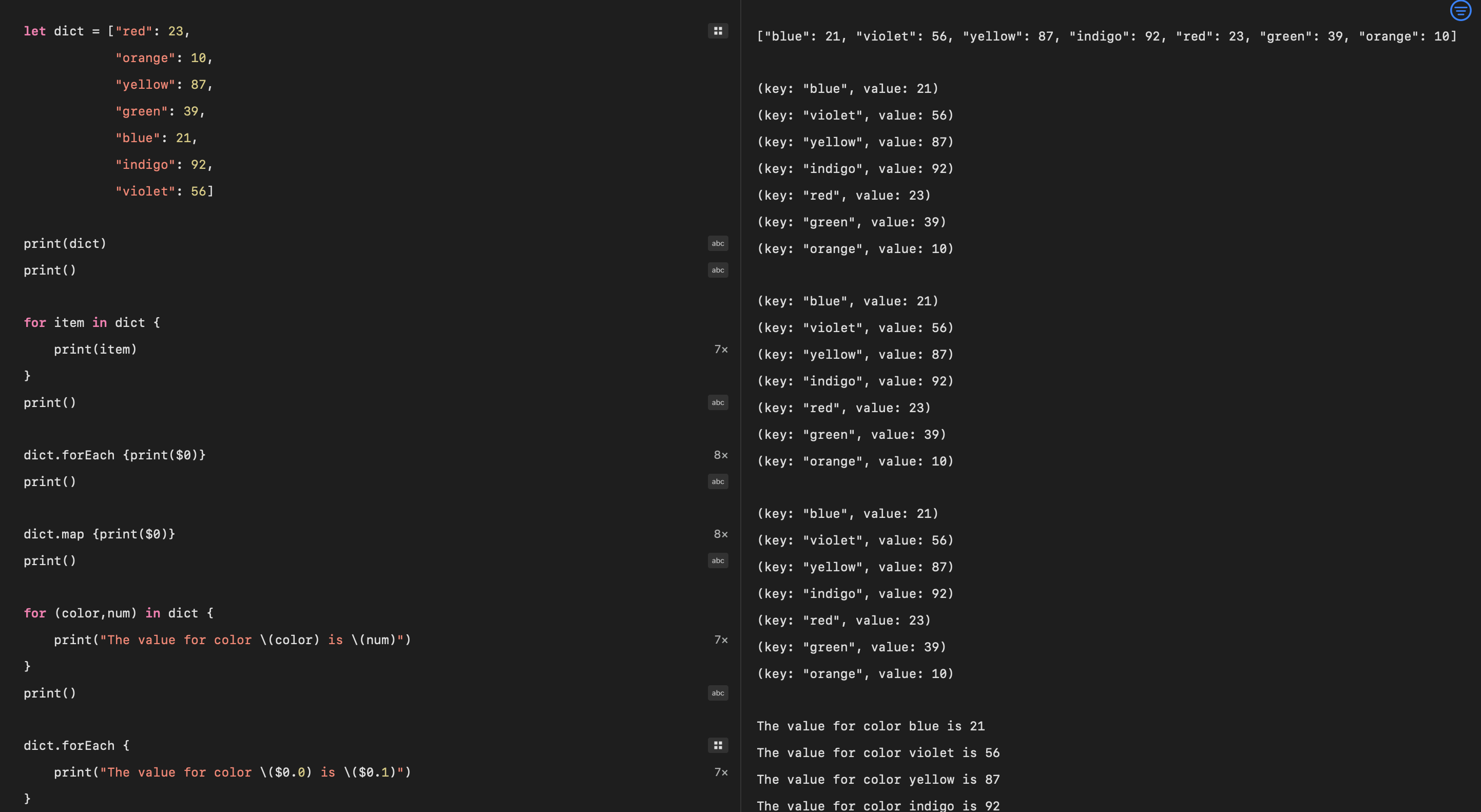For loops over dictionary in Swift playground

## Iterate on Keys and Values

It is possible to get a collection of either keys or values from a dictionary and iterate over these. This might be of limited value depending on the data in the dictionary.

 1let dict = ["red": 23,
2            "orange": 10,
3            "yellow": 87,
4            "green": 39,
5            "blue": 21,
6            "indigo": 92,
7            "violet": 56]
8
9for color in dict.keys {
10   print(color)
11}
12print()
13
14dict.keys.forEach {print($0)} 15print() 16 17dict.keys.map {print($0)}
18print()
19
20for num in dict.values {
21   print(num)
22}
23print()
24
25dict.values.forEach {print($0)} 26print() 27 28dict.values.map {print($0)}
29print()
30
31"""
32green
33indigo
34violet
35red
36blue
37orange
38yellow
39
40green
41indigo
42violet
43red
44blue
45orange
46yellow
47
48green
49indigo
50violet
51red
52blue
53orange
54yellow
55
5639
5792
5856
5923
6021
6110
6287
63
6439
6592
6656
6723
6821
6910
7087
71
7239
7392
7456
7523
7621
7710
7887
79"""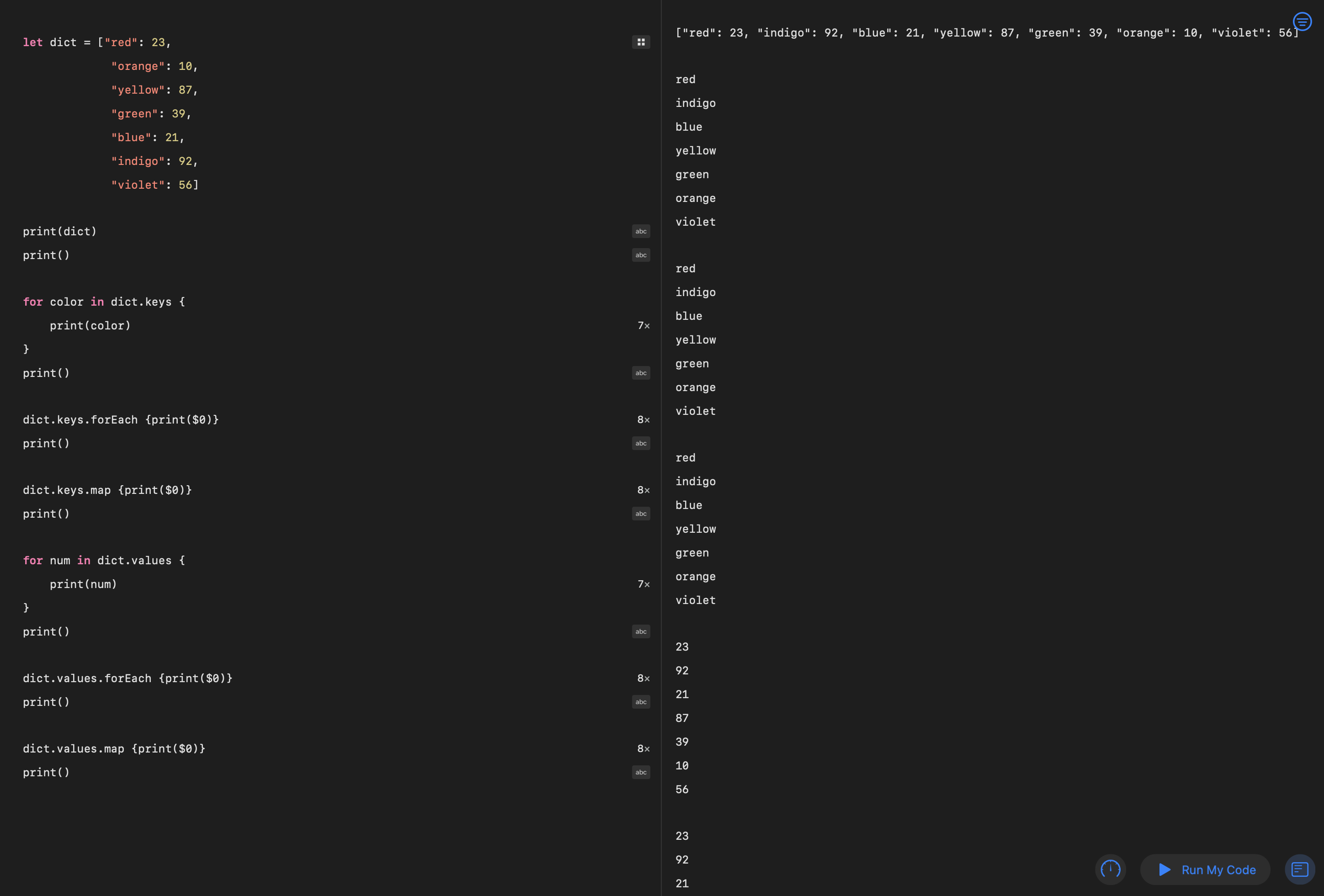Iterate over dictionary keys or values in Swift playground

## Enumerate on a Dictionary

The elements of a dictionary can be enumerated to return a sequence of pairs with the index as the first item and the dictionary key/value pair as the second element. The enumerated items are printed out as an offset and the dictionary element at that offset.

 1let dict = ["red": 23,
2           "orange": 10,
3           "yellow": 87,
4           "green": 39,
5           "blue": 21,
6           "indigo": 92,
7           "violet": 56]
8
9print(dict)
10print()
11
12for item in dict.enumerated() {
13   print(item)
14}
15print()
16
17"""
18["violet": 56, "blue": 21, "indigo": 92, "red": 23, "orange": 10, "yellow": 87, "green": 39]
19
20(offset: 0, element: (key: "violet", value: 56))
21(offset: 1, element: (key: "blue", value: 21))
22(offset: 2, element: (key: "indigo", value: 92))
23(offset: 3, element: (key: "red", value: 23))
24(offset: 4, element: (key: "orange", value: 10))
25(offset: 5, element: (key: "yellow", value: 87))
26(offset: 6, element: (key: "green", value: 39))
27
28"""

 1dict.enumerated().forEach {print($0)} 2print() 3 4dict.enumerated().map {print($0)}
5print()
6
7"""
8(offset: 0, element: (key: "violet", value: 56))
9(offset: 1, element: (key: "blue", value: 21))
10(offset: 2, element: (key: "indigo", value: 92))
11(offset: 3, element: (key: "red", value: 23))
12(offset: 4, element: (key: "orange", value: 10))
13(offset: 5, element: (key: "yellow", value: 87))
14(offset: 6, element: (key: "green", value: 39))
15
16(offset: 0, element: (key: "violet", value: 56))
17(offset: 1, element: (key: "blue", value: 21))
18(offset: 2, element: (key: "indigo", value: 92))
19(offset: 3, element: (key: "red", value: 23))
20(offset: 4, element: (key: "orange", value: 10))
21(offset: 5, element: (key: "yellow", value: 87))
22(offset: 6, element: (key: "green", value: 39))
23"""

 1for (i, (color,num)) in dict.enumerated() {
2   print("At index = \(i). The value for color \(color) is \(num)")
3}
4print()
5
6dict.enumerated().forEach {
7   print("At index = \($0). The value for color \($1.0) is \($1.1)") 8} 9print() 10 11dict.enumerated().map { 12 print("At index = \($0). The value for color \($1.0) is \($1.1)")
13}
14print()
15
16"""
17At index = 0. The value for color violet is 56
18At index = 1. The value for color blue is 21
19At index = 2. The value for color indigo is 92
20At index = 3. The value for color red is 23
21At index = 4. The value for color orange is 10
22At index = 5. The value for color yellow is 87
23At index = 6. The value for color green is 39
24
25At index = 0. The value for color violet is 56
26At index = 1. The value for color blue is 21
27At index = 2. The value for color indigo is 92
28At index = 3. The value for color red is 23
29At index = 4. The value for color orange is 10
30At index = 5. The value for color yellow is 87
31At index = 6. The value for color green is 39
32
33At index = 0. The value for color violet is 56
34At index = 1. The value for color blue is 21
35At index = 2. The value for color indigo is 92
36At index = 3. The value for color red is 23
37At index = 4. The value for color orange is 10
38At index = 5. The value for color yellow is 87
39At index = 6. The value for color green is 39
40"""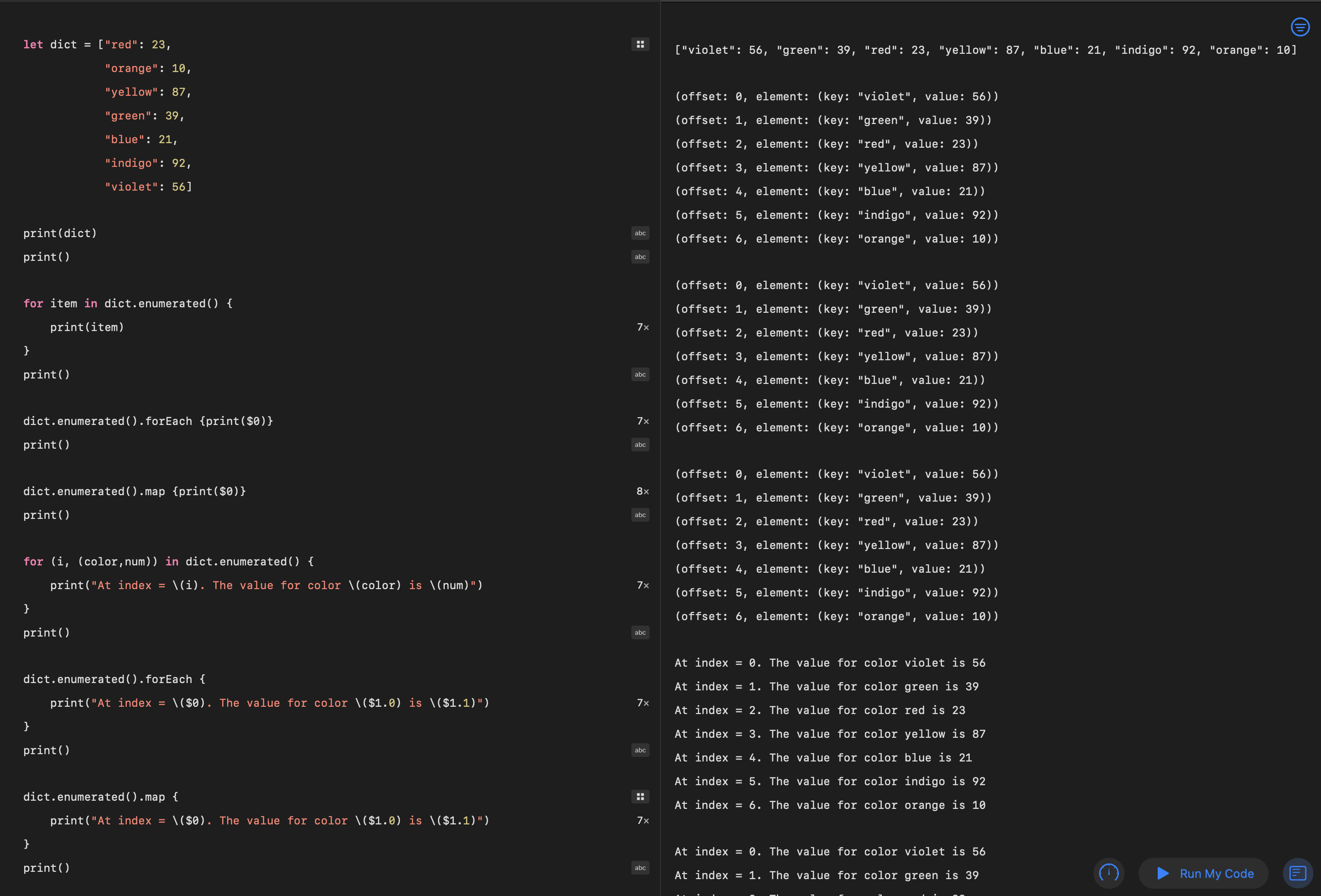Iterate over dictionary with enumerated in Swift playground

## Iterating on Sorted data in a Dictionary

Iterating over the elements in a dictionary becomes much more useful when the elements can be sorted. Although, this is no longer iterating over a dictionary as the sorted method returns an array of elements in sorted order from the dictionary. The default is to sort the elements by the dictionary keys. The more common requirement is to sort elements in a dictionary by their values and this is done using a closure specifying the dictionary values as the sorting predicate.

 1let dict = ["red": 23,
2           "orange": 10,
3           "yellow": 87,
4           "green": 39,
5           "blue": 21,
6           "indigo": 92,
7           "violet": 56]
8
9print(dict)
10print()
11
12"""
13["indigo": 92, "yellow": 87, "red": 23, "orange": 10, "green": 39, "violet": 56, "blue": 21]
14"""

 1print(dict.sorted(by: <))
2print()
3
4print(dict.sorted(by: >))
5print()
6
7"""
8[(key: "blue", value: 21), (key: "green", value: 39), (key: "indigo", value: 92), (key: "orange", value: 10), (key: "red", value: 23), (key: "violet", value: 56), (key: "yellow", value: 87)]
9
10[(key: "yellow", value: 87), (key: "violet", value: 56), (key: "red", value: 23), (key: "orange", value: 10), (key: "indigo", value: 92), (key: "green", value: 39), (key: "blue", value: 21)]
11"""

 1let sortedDict = dict.sorted { $0 <$1 }
2print(type(of: sortedDict))
3print()
4
5print(sortedDict)
6print()
7
8"""
9Array<(key: String, value: Int)>
10
11[(key: "blue", value: 21), (key: "green", value: 39), (key: "indigo", value: 92), (key: "orange", value: 10), (key: "red", value: 23), (key: "violet", value: 56), (key: "yellow", value: 87)]
12"""

 1sortedDict.forEach { print($0) } 2print() 3 4""" 5(key: "blue", value: 21) 6(key: "green", value: 39) 7(key: "indigo", value: 92) 8(key: "orange", value: 10) 9(key: "red", value: 23) 10(key: "violet", value: 56) 11(key: "yellow", value: 87) 12"""   1for (color,num) in sortedDict { 2 print("The value for color \(color) is \(num)") 3} 4print() 5 6sortedDict.forEach { 7 print("The value for color \($0.0) is \($0.1)") 8} 9print() 10 11""" 12The value for color blue is 21 13The value for color green is 39 14The value for color indigo is 92 15The value for color orange is 10 16The value for color red is 23 17The value for color violet is 56 18The value for color yellow is 87 19 20The value for color blue is 21 21The value for color green is 39 22The value for color indigo is 92 23The value for color orange is 10 24The value for color red is 23 25The value for color violet is 56 26The value for color yellow is 87   1let sortedDictValue = dict.sorted {$0.value > $1.value } 2print(sortedDictValue) 3print() 4 5print("Top 3 colors:") 6sortedDictValue.prefix(3).forEach { 7 print("Color \($0.0) is \($0.1)") 8} 9 10""" 11[(key: "indigo", value: 92), (key: "yellow", value: 87), (key: "violet", value: 56), (key: "green", value: 39), (key: "red", value: 23), (key: "blue", value: 21), (key: "orange", value: 10)] 12 13Top 3 colors: 14Color indigo is 92 15Color yellow is 87 16Color violet is 56 17"""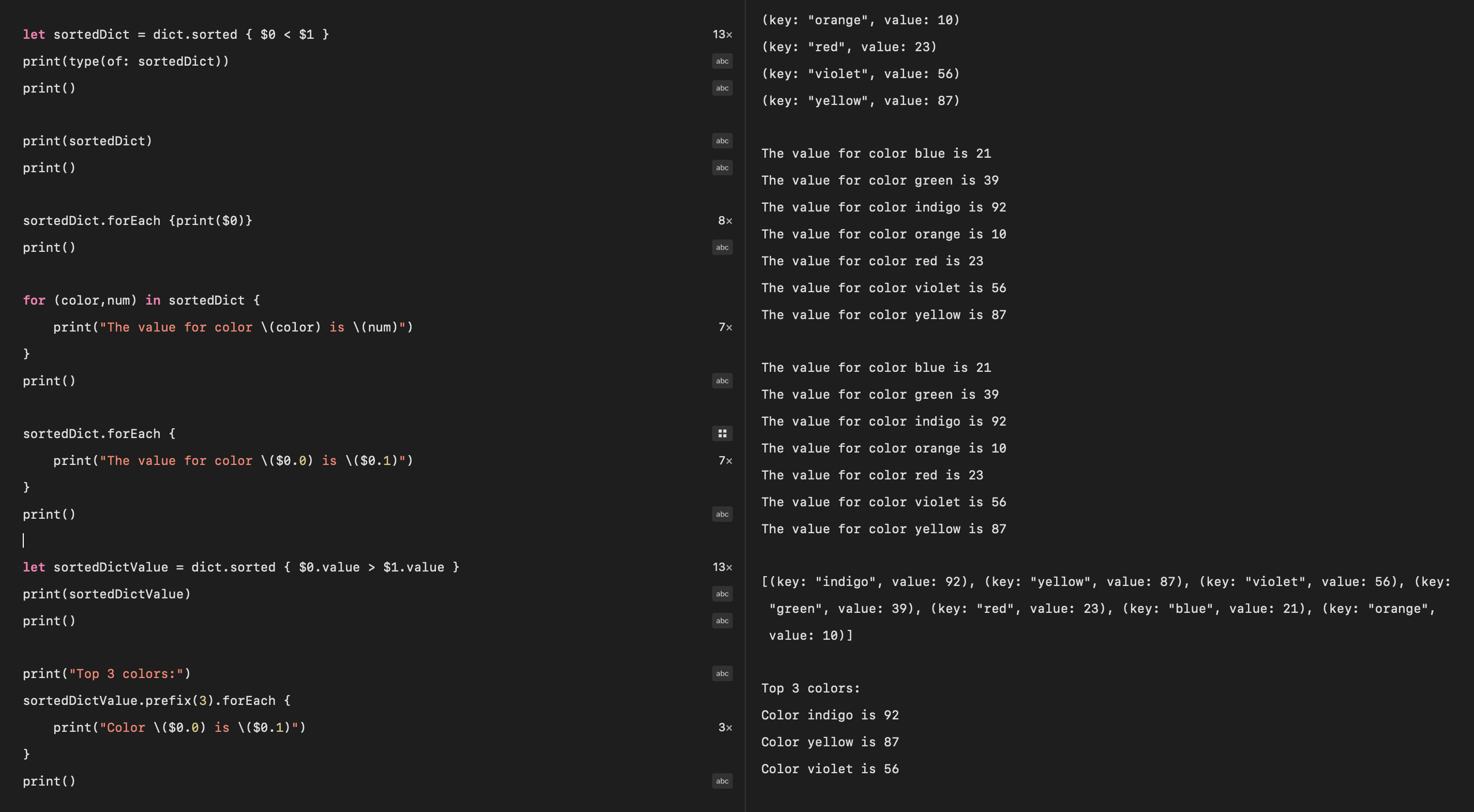Iterate over dictionary after sorting by key or value in Swift playground ## Get the top elements from Dictionary A combination of sorting the elements by value and returning the prefix number of elements can be used to retrieve the top x number of elements from a dictionary based on dictionary values. This is dependent on the element values conforming to Comparable protocol.  1let sortedDictValue = dict.sorted {$0.value > $1.value } 2print(sortedDictValue) 3print() 4 5print("Top 3 colors:") 6sortedDictValue.prefix(3).forEach { 7 print("Color \($0.0) is \($0.1)") 8} 9print() 10 11""" 12[(key: "indigo", value: 92), (key: "yellow", value: 87), (key: "violet", value: 56), (key: "green", value: 39), (key: "red", value: 23), (key: "blue", value: 21), (key: "orange", value: 10)] 13 14Top 3 colors: 15Color indigo is 92 16Color yellow is 87 17Color violet is 56 18"""  This code sorts the dictionary by decreasing values and prints out the top three, including their ranking number.  1dict.sorted {$0.value > $1.value } 2 .prefix(3) 3 .enumerated() 4 .forEach { print("\($0+1). \($1.0) = \($1.1)") }
5print()
6
7"""
81. indigo = 92
92. yellow = 87
103. violet = 56
11"""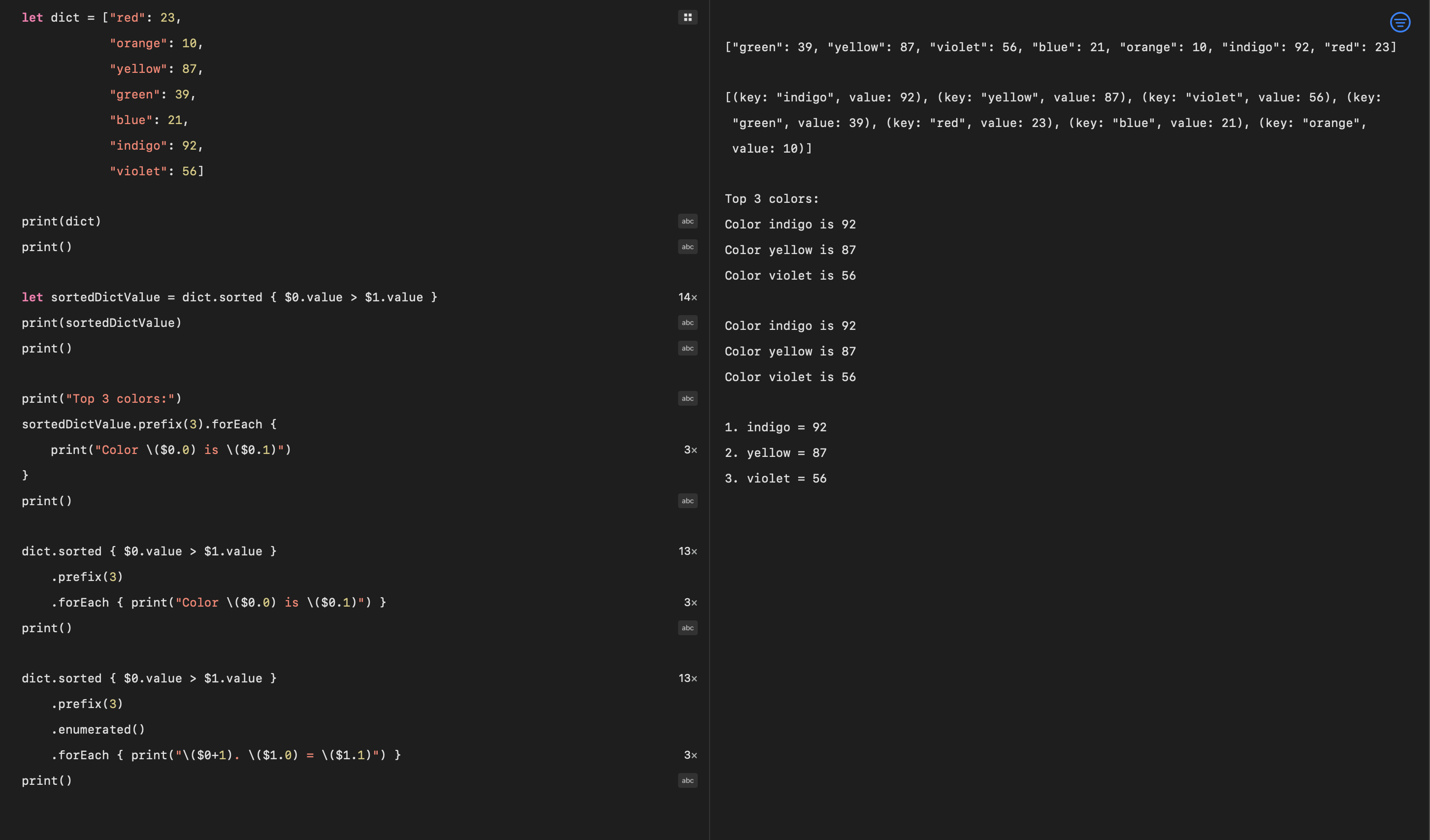Get the top 3 elements by value from a dictionary in Swift playground

## Conclusion

It is easy to iterate over a dictionary in swift using a for loop, a ForEach loop or a map function. The dictionary stores data in an unordered structure, so iterating may not yield the desired result. It is possible to apply the sorted method to the dictionary to give a sorted array of elements from the dictionary. The default is to sort the elements in a dictionary based on keys, but a trailing closure can be used to sort elements by value and the prefix method can be used to limit the number of elements returned.